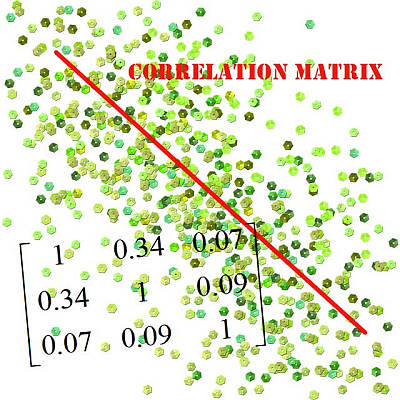# Correlation Matrix Calculator

Instructions: This correlation matrix calculator will provide you with a correlation matrix for a given set of samples. Please type in the box below two or more samples. Please press 'Enter' to start a new sample.Type the samples (comma or space separated, press 'Enter' for a new sample)Name of the samples (Separate with commas. Optional)

## More About the Correlation Matrix

A Correlation Matrix is a table in which the pairwise correlations between several variables are conveniently organized in a matrix form. The value in the ith row an jth column corresponds to the correlation between the variables $$X_i$$ and $$X_j$$.

In simple words, the correlation matrix is a summary of all the correlations that can be found for a set of variables, for which sample data is available.

Correlation calculation is crucial, because it is the previous step need to apply a linear regression calculator to find the Least Squares Model. But this should be attempted only when the correlation is found to be significant.## Correlation Matrix Formula

Since $$corr(X_i, X_j) = corr(X_j, X_i)$$, then the correlation matrix is symmetric, and for that reason, in order to not to be redundant, the correlation matrix only reports the values from the diagonal and up. For other correlation operations, you can compute a correlation coefficient showing all the steps, or you can use this critical correlation calculator .

## How do You compute a correlation Matrix

In order to understand how to compute a correlation matrix, you need to know first how to calculate Pearson's correlation, because the correlation matrix is simply the matrix of the correlations between all possible pairs of variables.

In order to proceed with the calculation of the correlation matrix, you need to follow these steps:

Step 1: List the variables you have, say X1, X2, ..., etc. Each of these variables has a sample associated to it

Step 2: Take the ith and jth variables from your list, Xi and Xj, and compute the correlation coefficient for them. Call it $$r_{ij}$$

Step 3: Take the value $$r_{ij}$$ and that will the value of the row i, column j of the correlation matrix

Correlation values close to 1 or -1 indicate strong linear associations, suggesting that a linear regression calculator (or a multiple linear regression, depending on how many predictors you have).